Courses

# Consumers’ and producers’ surplus - Integration, Business Mathematics & Statistics B Com Notes | EduRev

Created by: Arshit Thakur

## B Com : Consumers’ and producers’ surplus - Integration, Business Mathematics & Statistics B Com Notes | EduRev

The document Consumers’ and producers’ surplus - Integration, Business Mathematics & Statistics B Com Notes | EduRev is a part of the B Com Course Business Mathematics and Statistics.
All you need of B Com at this link: B Com

CONSUMERS’ SURPLUS
A demand curve for a commodity shows the amount of the commodity that will be bought by people at any given price p.

Suppose that the prevailing market price is p0 . At this price an amount x0 of the commodity determined by the demand curve will be sold. However there are buyers who would be willing to pay a price higher than p0 . All such buyers will gain from the fact that the prevailing market price is only p0 . This gain is called Consumers’ Surplus . It is represented by the area below the demand curve p = f(x) and above the line p = p0 . Thus Consumers’ Surplus, CS = [Total area under the demand function bounded by x = 0, x = x0 and x-axis - Area of the rectangle OAPB]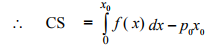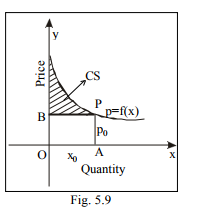Example 26 Fig. 5.9 Find the consumers’ surplus for the demand function p = 25 - x - x2 when p = 19.
Solution :
Given that, The demand function is p = 25 - x - x2 p0 = 19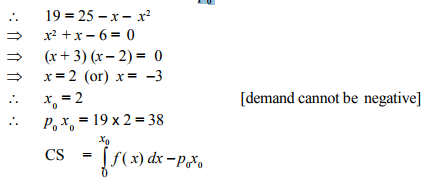Example 27
The demand of a commodity is p = 28 - x 2 Find the consumers’ surplus when demand x0 = 5

Solution : Given that,
The demand function, p = 28 - x 2
when x0 = 5 p0 = 28 - 25
= 3
∴  p0 x0 = 15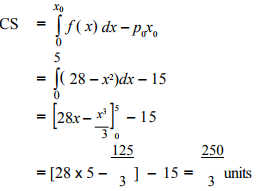Example 28
The demand function for a commodity is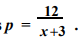. Find the consumers’ surplus when the prevailing market price is 2.

Solution : Given that, Demand function,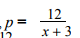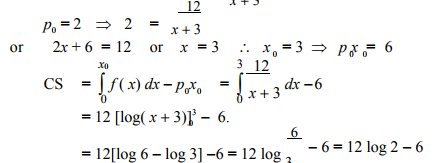PRODUCERS’ SURPLUS
A supply curve for a commodity shows the amount of the commodity that will be brought into the market at any given pricep. Suppose the prevailing market price is p0 . At this price an amount x0 of the commodity, determined by the supply curve, will be offered to buyers. However, there are producers who are willing to supply the commodity at a price lower than p0 . All such producers will gain from the fact that the prevailing market price is only p0 . This gain is called ‘Producers’ Surplus’. It is represented by the area above the supply curve p = g(x ) and below the line p = p0 . Thus Producers’ Surplus, PS = [ Area of the whole rectangle OAPB  Area under the supply curve bounded by x = 0, x = x0 and x - axis]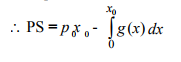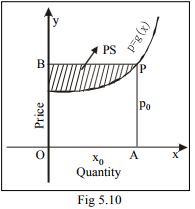Example 29
The supply function for a commodity is p = x2 + 4x + 5 where x denotes supply. Find the producers’ surplus when the price is 10.

Solution : Given that, Supply function, p = x 2 + 4x + 5
For p0 = 10,
10 = x 2 + 4x + 5 + x 2 + 4x + 5 = 0
⇒ (x + 5) (x + 1) = 0 + x = + 5 or x = 1

Since supply cannot be negative, x = + 5 is not possible.

∴  x = 1
∴ p = 10 and x0  = 1
⇒  p0xP0 = 10

Producers’ Surplus,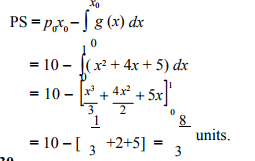Example 30  Find the producers’ surplus for the supply function p = x2 + x + 3 when x = 4.
Solution :
Given that,
supply function p = x 2 + x + 3

when x0 = 4, p0 = 4 + 4 +3 = 23
∴ p x = 92.

Producers’ Surplus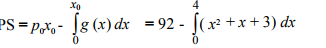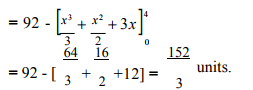Example 31  Find the producers’ surplus for the supply function p = 3 + x2 when the price is 12.

Solution :
Given that,
supply function, p = 3 + x2 .  When p0 = 12,
12 = 3 + x2 or x2 = 9 or x = + 3

Since supply cannot be negative,
12 = 3 + x2 or x 2 = 9 or x = + 3

Since supply cannot be negative

x = 3. i.e. x0 = 3,

∴ p x = 36.

Producers’ Surplus,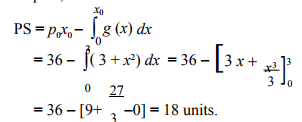Example 32

The demand and supply functions under pure competition are pd = 16 - x and ps = 2x + 4. Find the consumers’ surplus and producers’ surplus at the market equilibrium price.

Solution : For market equilibrium, Quantity demanded = Quantity supplied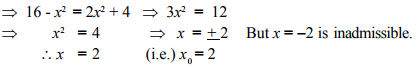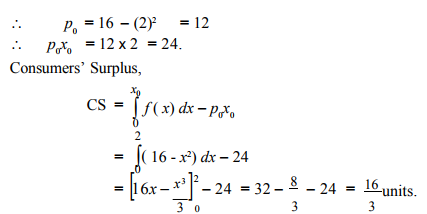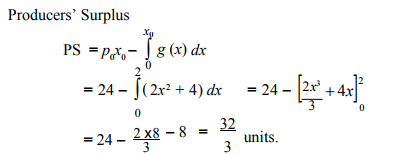122 videos|142 docs

,

,

,

,

,

,

,

,

,

,

,

,

,

,

,

,

,

,

,

,

,

,

,

,

;